# Line segment

Cut a line segment of 15 cm into two line segments so that their lengths are in ratio 2:1. What length will each have?

a =  10 cm
b =  5 cm

### Step-by-step explanation:

a+b = 15
a = 2b

a+b = 15
a = 2•b

a+b = 15
a-2b = 0

a = 10
b = 5

Our linear equations calculator calculates it.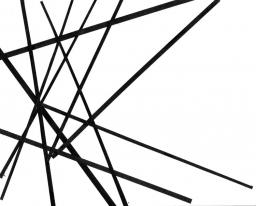Did you find an error or inaccuracy? Feel free to write us. Thank you!Tips to related online calculators
Check out our ratio calculator.
Do you have a linear equation or system of equations and looking for its solution? Or do you have a quadratic equation?

## Related math problems and questions:

• Line segmentThe 4 cm long line segment is enlarged in the ratio of 5/2. How many centimeters will measure the new line segment?
• Line segment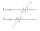Line segment AB is 8 cm long. Divide it in a ratio of 2: 3.
• A ropeA rope can be cut into equal length with no rope left over. The lengths can be 15cm,18cm or 25cm. What is the shortest possible length of the rope?
• Divide in ratioLine segment AB 12 cm long divide in a ratio of 5: 3. How long are the individual parts?
• Points on line segmentPoints P & Q belong to segment AB. If AB=a, AP = 2PQ = 2QB, find the distance: between point A and the midpoint of the segment QB.
• SegmentsLine segments 62 cm and 2.2 dm long we divide into equal parts which lengths in centimeters is expressed integer. How many ways can we divide?
• Map 2At what scale is made map if the distance 8.2 km corresponds on the map segment 5 cm long?
• MG=7x-15,Length of lines MG = 7x-15 and FG = 33 Point M is the midpoint of FG. Find the unknown x.
• Sides od triangleSides of the triangle ABC has length 4 cm, 5 cm and 7 cm. Construct triangle A'B'C' that are similar to triangle ABC which has a circumference of 12 cm.
• What scale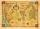What scale is the map drawn if it shows a 15km route from the station to the ruins with a line 30cm long? A) 1: 2000 B) 1: 5000 C) 1: 20,000 D) 1: 50,000
• CuboidThe sum of the lengths of the three edges of the cuboid that originate from one vertex is 210 cm. Edge length ratio is 7: 5: 3. Calculate the length of the edges.
• The scaleThe distance of 15 cm on the map corresponds to a distance of 27 km in reality. What is the scale of the map?
• SegmentsWhich of the pairs of numbers on the number line encloses the longest segment: ?
• 2d shapeCalculate the content of a shape in which an arbitrary point is not more than 3 cm from the segment AB. The length of the segment AB is 5 cm.
• Cuboid face diagonalsThe lengths of the cuboid edges are in the ratio 1: 2: 3. Will the lengths of its diagonals be the same ratio? The cuboid has dimensions of 5 cm, 10 cm, and 15 cm. Calculate the size of the wall diagonals of this cuboid.
• The string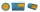They cut 113 cm from the string and divided the rest in a ratio of 5: 6.5: 8: 9.5. The longest part measured 38 cm. Find the original length of the string.
• Dividing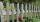Divide the three-line segment 13 cm, 26 cm, and 19.5 cm long for parts so that the individual pieces were equally long and longest. How long will the individual parts, and how many will it?